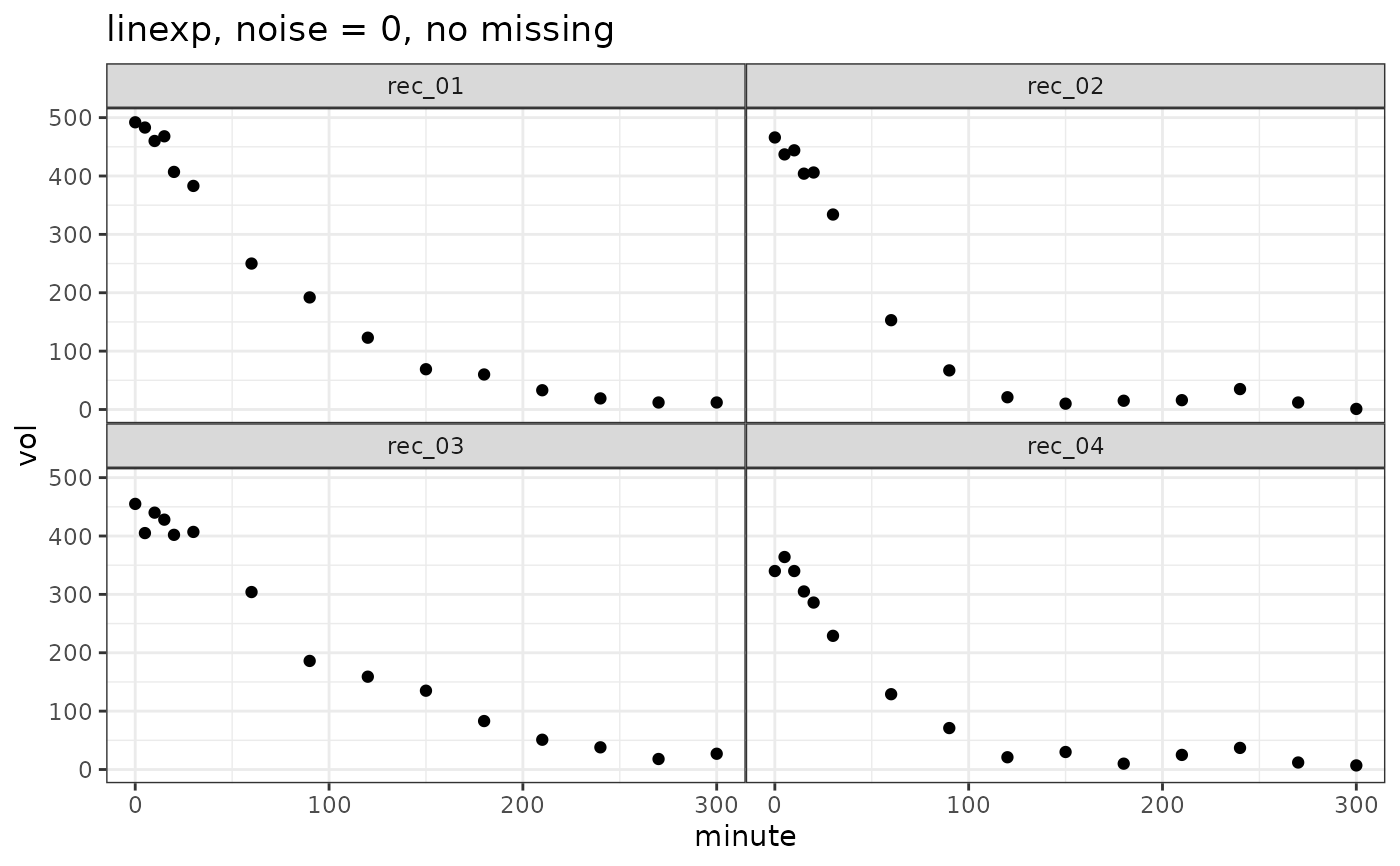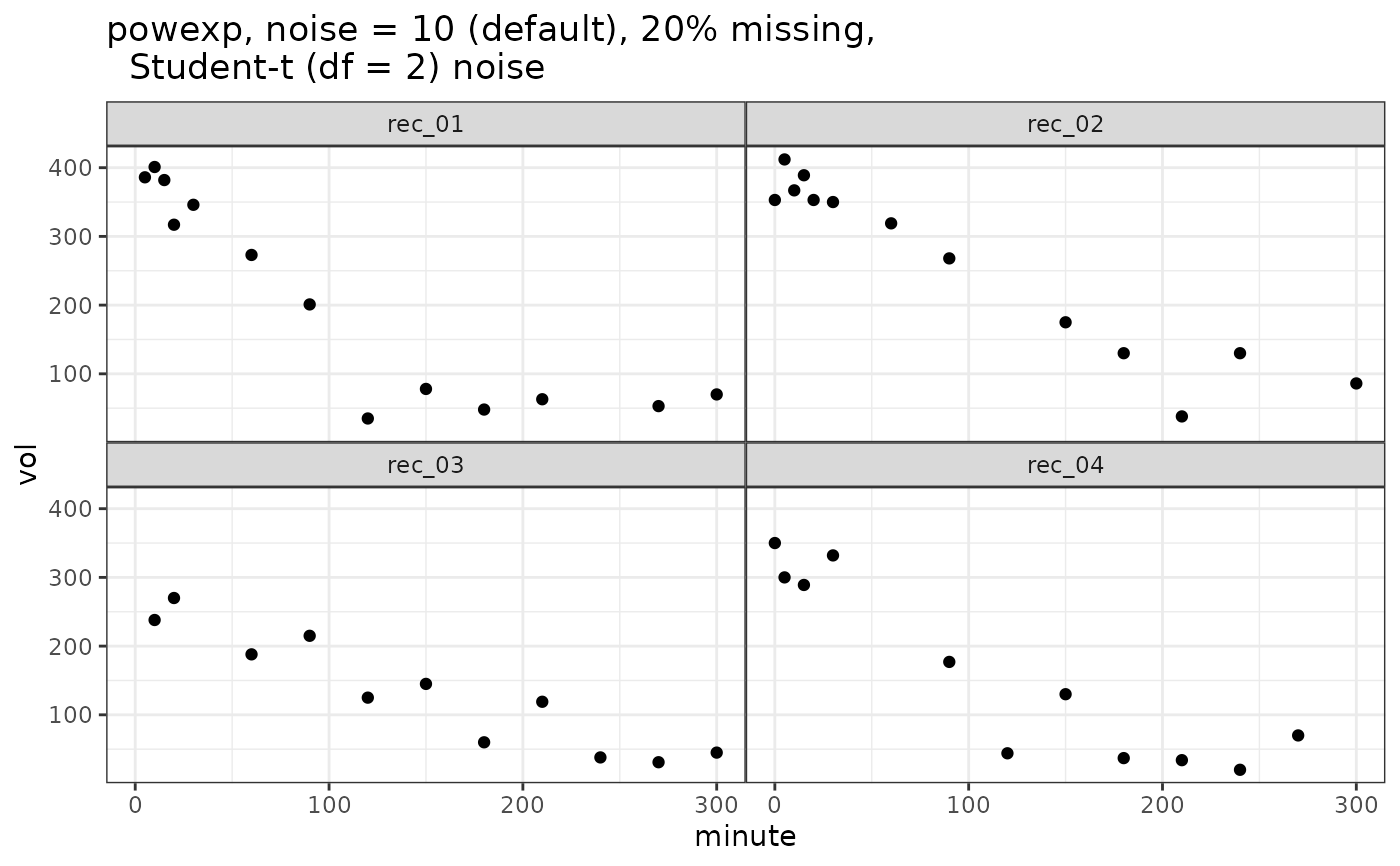Simulate gastric emptying data following a linexp or powexp function

## Usage

simulate_gastempt(
n_records = 10,
v0_mean = 400,
v0_std = 50,
tempt_mean = ifelse(identical(model, linexp), 60, 120),
tempt_std = tempt_mean/3,
kappa_mean = 0.7,
kappa_std = kappa_mean/3,
beta_mean = 0.7,
beta_std = beta_mean/3,
noise = 20,
student_t_df = NULL,
missing = 0,
model = linexp,
seed = NULL,
max_minute = NULL
)

## Arguments

n_records

Number of records

v0_mean, v0_std

Mean and between record standard deviation of initial volume, typically in ml.

tempt_mean, tempt_std

Mean and between record standard deviation of parameter $t_empt$, typically in minutes.

kappa_mean, kappa_std

For linexp only: Mean and between-record standard deviation of overshoot parameter kappa. For values of kappa above 1, curve has an overshoot that can be used to follow volume time series with secretion.

beta_mean, beta_std

For powexp only: Mean and between-record standard deviation of the so called lag parameter.

noise

Standard deviation of normal noise when student_t_df = NULL; scaling of noise when student_t_df >= 2.

student_t_df

When NULL (default), Gaussian noise is added; when >= 2, Student_t distributed noise is added, which generates more realistic outliers. Values from 2 to 5 are useful, when higher values are used the result comes close to that of Gaussian noise. Values below 2 are rounded to 2.

missing

When 0 (default), all curves have the same number of data points. When > 0, this is the fraction of points that were removed randomly to simulate missing points. Maximum value is 0.5.

model

linexp(default) or powexp

seed

optional seed; not set if seed = NULL (default)

max_minute

Maximal time in minutes; if NULL, a sensible default rounded to hours is used

## Value

A list with 3 elements:

record

Data frame with columns record(chr), v0, tempt, kappa/beta giving the effective linexp or powexp parameters for the individual record. v0 is rounded to nearest integer.

data

Data frame with columns record(chr), minute(dbl), vol(dbl) giving the time series and grouping parameters. vol is rounded to nearest integer.

stan_data

A list for use as data in Stan-based fits with elements prior_v0, n, n_record, record, minute, volume.

A comment is attached to the return value that can be used as a title

## Examples

suppressWarnings(RNGversion("3.5.0"))
set.seed(4711)
library(ggplot2)
vol_linexp = simulate_gastempt(n_records = 4, noise = 20)
ggplot(vol_linexp$data, aes(x = minute, y = vol)) + geom_point() + facet_wrap(~record) + ggtitle("linexp, noise = 0, no missing")vol_powexp = simulate_gastempt(n_records = 4, missing = 0.2, student_t_df = 2) ggplot(vol_powexp$data, aes(x = minute, y = vol)) + geom_point() +
facet_wrap(~record) + ggtitle("powexp, noise = 10 (default), 20% missing,
Student-t (df = 2) noise")